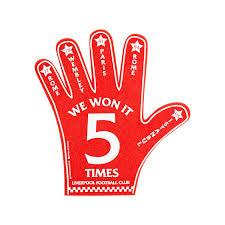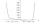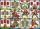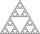# Value

Find the value of the expression:

6!·10^-3

Correct result:

x =  0.72

#### Solution:We would be pleased if you find an error in the word problem, spelling mistakes, or inaccuracies and send it to us. Thank you!## Next similar math problems:

• EquationEquation ? has one root x1 = 8. Determine the coefficient b and the second root x2.
• RootsDetermine the quadratic equation absolute coefficient q, that the equation has a real double root and the root x calculate: ?
• Calculation of CNCalculate: ?
• Median and modusRadka made 50 throws with a dice. The table saw fit individual dice's wall frequency: Wall Number: 1 2 3 4 5 6 frequency: 8 7 5 11 6 13 Calculate the modus and median of the wall numbers that Radka fell.
• SeatsSeats in the sport hall are organized so that each subsequent row has five more seats. First has 10 seats. How many seats are: a) in the eighth row b) in the eighteenth row
• Unknown numberI think number. If subtract from the twelfth square the ninth square I get a number 27 times greater than the intended number. What is this unknown number?
• Five membersWrite first 5 members geometric sequence and determine whether it is increasing or decreasing: a1 = 3 q = -2
• Tenth memberCalculate the tenth member of geometric sequence when given: a1=1/2 and q=2
• GP - 8 itemsDetermine the first eight members of a geometric progression if a9=512, q=2
• CalculationHow much is sum of square root of six and the square root of 225?
• Geometric sequence 4It is given geometric sequence a3 = 7 and a12 = 3. Calculate s23 (= sum of the first 23 members of the sequence).
• Sequence 3Write the first 5 members of an arithmetic sequence: a4=-35, a11=-105.
• CardsHow many ways can give away 32 playing cards to 7 player?
• Permutations without repetitionFrom how many elements we can create 720 permutations without repetition?
• AS sequenceIn an arithmetic sequence is given the difference d = -3 and a71 = 455. a) Determine the value of a62 b) Determine the sum of 71 members.
• Big factorialHow many zeros end number 116! ?
• PowerNumber ?. Find the value of x.